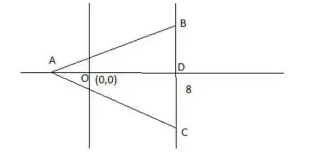### CAT 2018 – Slot 2 – Quantitative Ability – A triangle ABC has area 32 sq units

Q. 1: A triangle ABC has area 32 sq units and its side BC, of length 8 units, lies on the line x = 4. Then the shortest possible distance between A and the point (0,0) is
1. 4 units
2. 8 units
3. 4√2 units
4. 2√2 units

The distance OA will be minimum when the perpendicular from A on BC will pass through O.Area of triangle ABC = 1/2 BC×AD = 1/2×8×(AO+OD ) = 4×(AO+4)

32 = 4AO+16

AO = 4

So required minimum distance = 4

##### Q. 2: There are two drums, each containing a mixture of paints A and B. In drum 1, A and B are in the ratio 18 : 7. The mixtures from drums 1 and 2 are mixed in the ratio 3 : 4 and in this final mixture, A and B are in the ratio 13 : 7. In drum 2, then A and B were in the ratio1. 229 : 1412. 220 : 1493. 239 : 1614. 251 : 163

Let in drum 2, A and B are in the ratio m : n.

Given, In drum 1, A and B were in the ratio 18 : 7 = 18x : 7x .

Final mixtures ratio of contribution of drum 1 and drum 2 = 3: 4 = 75x : 100x

So A in final mixture = 18x × 3+100x × m/(m+n) = ( 154m+54n)/(m+n) x

B in final mixture = 7x × 3+100x × n/(m+n) = ( 21m+121n)/(m+n) x

Given A : B in final mixture = 13:7

Or (154m+54n )/(21m+121n )=13/7

1078m+378n=273m+1573n

805m=1195n

m:n=1195∶805=239∶161

Q. 3: Points A, P, Q and B lie on the same line such that P, Q and B are, respectively, 100 km, 200 km and 300 km away from A. Cars 1 and 2 leave A at the same time and move towards B. Simultaneously, car 3 leaves B and moves towards A. Car 3 meets car 1 at Q, and car 2 at P. If each car is moving in uniform speed then the ratio of the speed of car 2 to that of car 1 is
1. 1 : 2
2. 2 : 9
3. 1 : 4
4. 2 : 7

Car 3 meets car 1 at Q, which is 200 km from A.

Therefore, at the time of their meeting car 1 must have travelled 200 km and car 3 must have travelled 100 km.

As the time is same, ratio of speed of car 1 to speed of car 3 = 2 : 1. Car 3 meets car 2 at P, which is 100 km from A.

Therefore, at the time of their meeting car 2 must have travelled 100 km and car 3 must have travelled 200 km.

As the time is same, ratio of speed of car 2 to speed of car 3 = 1 : 2. Speed of car 1 : speed of car 3 = 2 : 1

And speed of car 2 : speed of car 3 = 1 : 2

So, speed of car 1 : speed of car 2 : speed of car 3 = 4 : 1 : 2

Q. 4:1/log(base2⁡)100 -1/log(base4)⁡100 +1/log(base5)⁡100 -1/log(base10)⁡100 +1/log(base20⁡)100 -1/log(base25)⁡100 +1/log(base50)⁡100 —?
1. 1/2
2. 0
3. 10
4. −4

Using log(basea)⁡b = 1/log(baseb⁡)a

1/log(base2⁡)100 -1/log(base4⁡)100 +1/log(base5⁡)100 -1/log(base10)⁡100 +1/log(base20)⁡100 -1/log(base25)⁡100 +1/log(base50)⁡100

= log(base100)⁡2- log(base100)⁡4+ log(base100⁡)5-log(base100⁡)10+ log(base100⁡)20-log(base100⁡)25+log(base100⁡)50

=log(base100)⁡ (2×5×20×50)/(4×10×25)

=log(base(10^2 ))⁡10=1/2

##### Checkout Other Questions of CAT 2018 Slot 2 Paper:

Verbal Ability :              |   Q.01- Q.05  |  Q.06- Q.9  |  Q.10- Q.14  |  Q.15- Q.19  |  Q.20- Q.24  |  Q.25- Q.29  |  Q.30 – Q.34  |

Logical Reasoning :    |   Q.29 – Q.32  |

Quantitative Aptitude: |